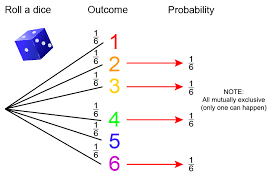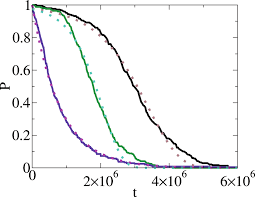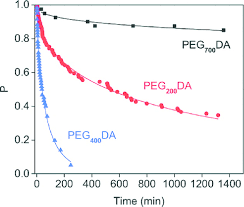## How to Calculate and Solve for Mutually Non-Exclusive | ProbabilityThe image above represents mutually non-exclusive.

To compute for mutually non-exclusive, four essential parameters are needed and these parameters are xA, NA, xB and NB.

The formula for calculating mutually non-exclusive:

P(A or B) = P(A) + P(B) – P(A and B)

Where;

P(A or B) = Mutually Non-Exclusive
P(A) = xANA
P(B) = xBNB

Let’s solve an example;
Find the mutually non-exclusive when the xA is 10, NA is 20, xB is 5 and NB is 12.

This implies that;

xA = 10
NA = 20
xB = 5
NB = 12

P(A or B) = P(A) + P(B) – P(A and B)
P(A or B) = P(A) + P(B) – (P(A) x P(B))
P(A or B) = xANA + xBNB – (xANA x xB NB)
P(A or B) = 10 20 + 5 12 – (10 20 x 5 12)
P(A or B) = 10(12) + 5(20) (20)(12) – ((10)(5) (20)(12))
P(A or B) = 120 + 100 240 – (50 240)
P(A or B) = 220 240 – 50 240
P(A or B) = (220 – 50) 240
P(A or B) = 170 240
Dividing the numerator and denominator by 10
P(A or B) = 17 24
P(A or B) = 0.708

Therefore, the mutually non-exclusive is 0.708.

## How to Calculate and Solve for Mutually Exclusive | ProbabilityThe image above represents mutually exclusive.

To compute for mutually exclusive, four essential parameters are needed and these are xA, NA, xB and NB.

The formula for calculating mutually exclusive:

P(A or B) = P(A) + P(B)

Where;

P(A or B) = Mutually Exclusive
P(A) = xANA
P(B) = xBNB

Let’s solve an example;
Find the mutually exclusive when the xA is 12, NA is 14, xB is 9 and NB is 17.

This implies that;

xA = 12
NA = 14
xB = 9
NB = 17

P(A or B) = P(A) + P(B)
P(A or B) = xANA + xBNB
P(A or B) = 1214 + 917
P(A or B) = 12(17) + 9(14)(14)(17)
P(A or B) = 204 + 126238
P(A or B) = 330238
Dividing the numerator and denominator by 2
P(A or B) = 165119
P(A or B) = 1.386

Therefore, the mutually exclusive is 1.386.

## How to Calculate and Solve for P(A and B) | ProbabilityThe image above represents P(A and B).

To compute for P(A and B), four essential parameters are needed and these parameters are xA, NA, xB and NB.

The formula for calculating P(A and B):

P(A and B) = P(A) x P(B)

Where;

P(A) =  xANA
P(B) = xBNB

Let’s solve an example;
Find the P(A and B) when xA is 2, NA is 4, xB is 5 and NB is 8.

This implies that;

xA = 2
NA = 4
xB = 5
NB = 8

P(A and B) = P(A) x P(B)
P(A and B) = xANA x xBNB
P(A and B) = 24 x 58
P(A and B) = (2)(5)(4)(8)
P(A and B) = 1032
Divinding the numerator and denominator by 2
P(A and B) = 516
P(A and B) = 0.312

Therefore, the P(A and B) is 0.312.

## How to Calculate and Solve for P(A or B) | ProbabilityThe image above represents P(A or B).

To compute for P(A or B), four essential parameters are needed and these parameters are xA, NA, xB and NB.

The formula for calculating P(A or B):

P(A or B) = P(A) + P(B)

Where;

P(A) =  xANA
P(B) = xBNB

Let’s solve an example;
Find the P(A or B) when xA is 9, NA is 11, xB is 6 and NB is 12.

This implies that;

xA = 9
NA = 11
xB = 6
NB = 12

P(A or B) = P(A) + P(B)
P(A or B) = xANA + xBNB
P(A or B) = 911 + 612
P(A or B) = 9(12) + 6(11)(11)(12)
P(A or B) = 108 + 66132
P(A or B) = 174132
Dividing the numerator and denominator by 6
P(A or B) = 2922
P(A or B) = 1.318

Therefore, the P(A or B) is 1.318.